# Workers at a certain soda drink factory collected data on the volumes (in ounces) of simple...

Workers at a certain soda drink factory collected data on the volumes (in ounces) of simple random sample of 15 cans of the soda drink Those volumes have mean of 12.19 oz and a standard deviation of 0.14 oz and they appear be from a normally distributed population. If the workers Want the filling process to work so that almost all cans have volume between 11.88 and 12.52, and the standard deviation should be less than 0.16 oz. use the sample data test that claim that the population of volumes has a standard deviation less than 0.16. Use a 0.025 significance level. B) compute the test statistic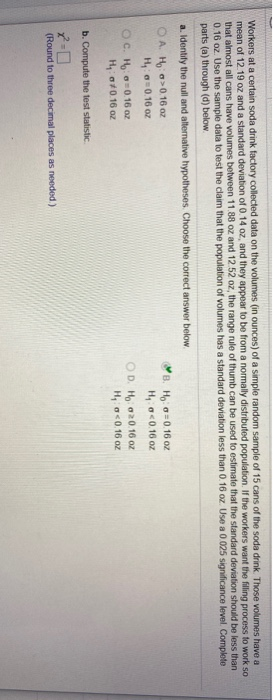Workers at a certain soda drink factory collected data on the volumes (in ounces) of a simple random sample of 15 cans of the soda drink. Those volumes have a mean of 12.19 oz and a standard deviation of 0.14 oz, and they appear to be from a normally distributed population. If the workers want the filling process to work so that almost all cans have volumes between 11.88 oz and 12 52 oz, the range rule of thumb can be used to estimate that the standard deviation should be less than 0.16 oz. Use the sample data to test the claim that the population of volumes has a standard deviation less than 0.16 oz. Use a 0.025 significance level. Complete parts (a) through (d) below a. Identify the null and alternative hypotheses. Choose the correct answer below. O A. Ho > 0.16 oz B. HO0.16 oz H0.16 02 Ho<0.16 oz OCH 0.16 02 OD. H 2016 2 H, 670.16 oz Ho<0.16 oz b. Compute the lost statistic (Round to three decimal places as needed)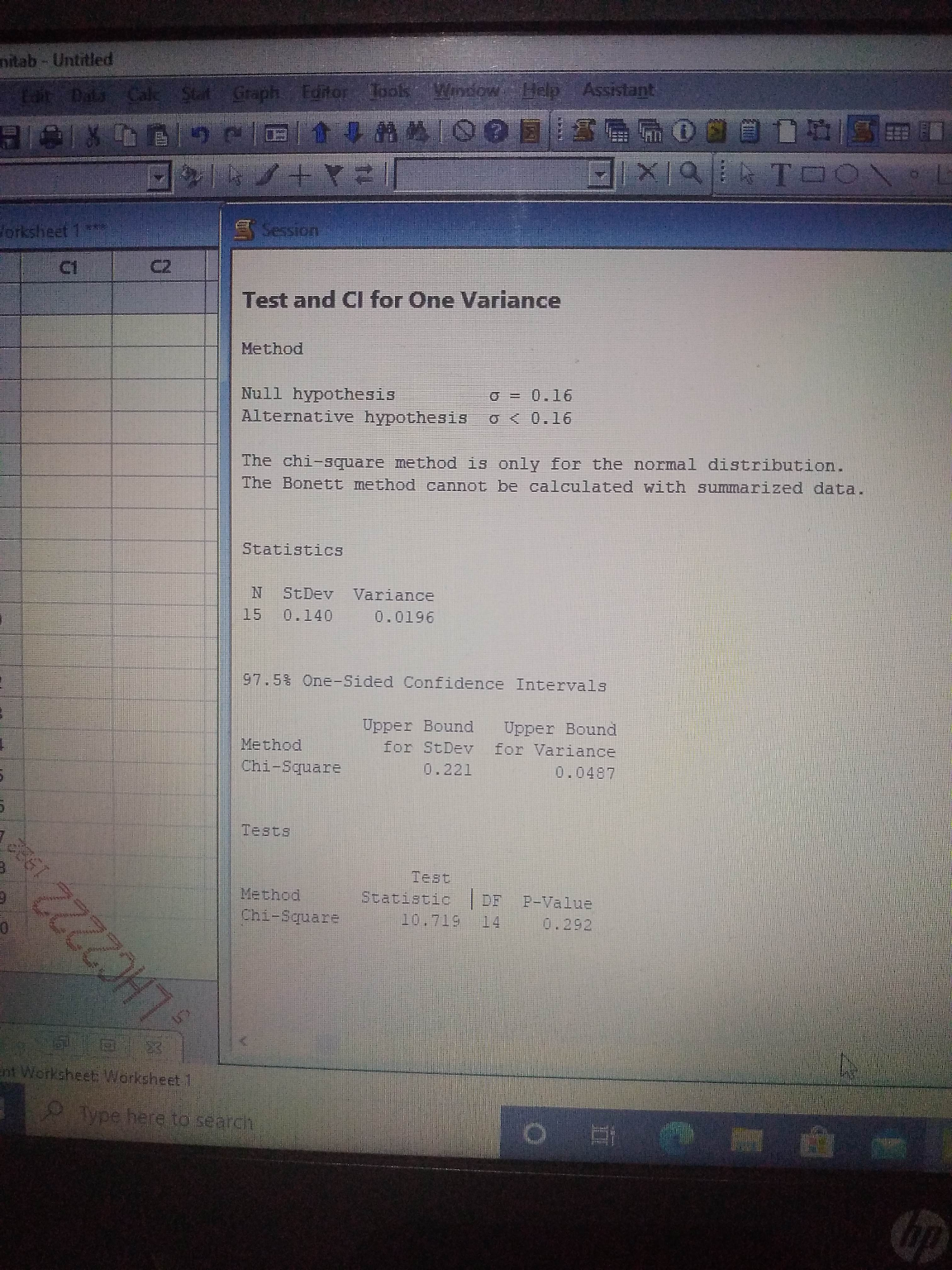Test statistics = 10.719

Dear student,
Thank You

##### Add Answer of: Workers at a certain soda drink factory collected data on the volumes (in ounces) of simple...
Similar Homework Help Questions
• ### Workers at a certain soda drink factory collected data on the volumes (in ounces) of simple...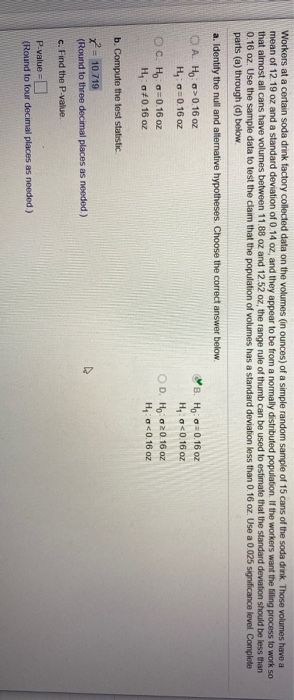Workers at a certain soda drink factory collected data on the volumes (in ounces) of simple random sample of 15 cans of the soda drink Those volumes have mean of 12.19 oz and a standard deviation of 0.14 oz and they appear be from a normally distributed population. If the workers Want the filling process to work so that almost all cans have volume between 11.88 and 12.52, and the standard deviation should be less than 0.16 oz. use the...

• ### Question Help Workers at a certain soda drink factory collected data on the volumes​ (in ounces)...

Question Help Workers at a certain soda drink factory collected data on the volumes​ (in ounces) of a simple random sample of 21 cans of the soda drink. Those volumes have a mean of 12.19 oz and a standard deviation of 0.09 ​oz, and they appear to be from a normally distributed population. If the workers want the filling process to work so that almost all cans have volumes between 11.95 oz and 12.59 ​oz, the range rule of thumb...

• ### Workers at a certain soda drink factory collected data on the volumes​ (in ounces) of a...

Workers at a certain soda drink factory collected data on the volumes​ (in ounces) of a simple random sample of 24 cans of the soda drink. Those volumes have a mean of 12.19 oz and a standard deviation of 0.09 ​oz, and they appear to be from a normally distributed population. If the workers want the filling process to work so that almost all cans have volumes between 12.04 oz and 12.56 ​oz, the range rule of thumb can be...

• ### Workers at a certain soda drink factory collected data on the volumes (in ounces) of a...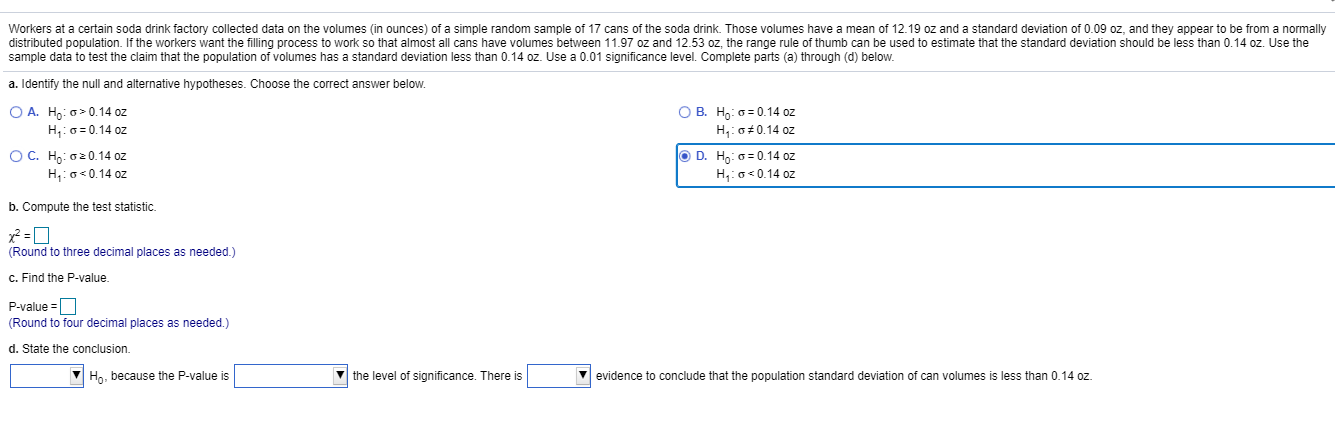Workers at a certain soda drink factory collected data on the volumes (in ounces) of a simple random sample of 17 cans of the soda drink. Those volumes have a mean of 12.19 oz and a standard deviation of 0.09 oz, and they appear to be from a normally distributed population. If the workers want the filling process to work so that almost all cans have volumes between 11.97 oz and 12.53 oz, the range rule of thumb can be...

• ### Workers at a certain soda drink factory collected data on the volumes (in ounces) of a...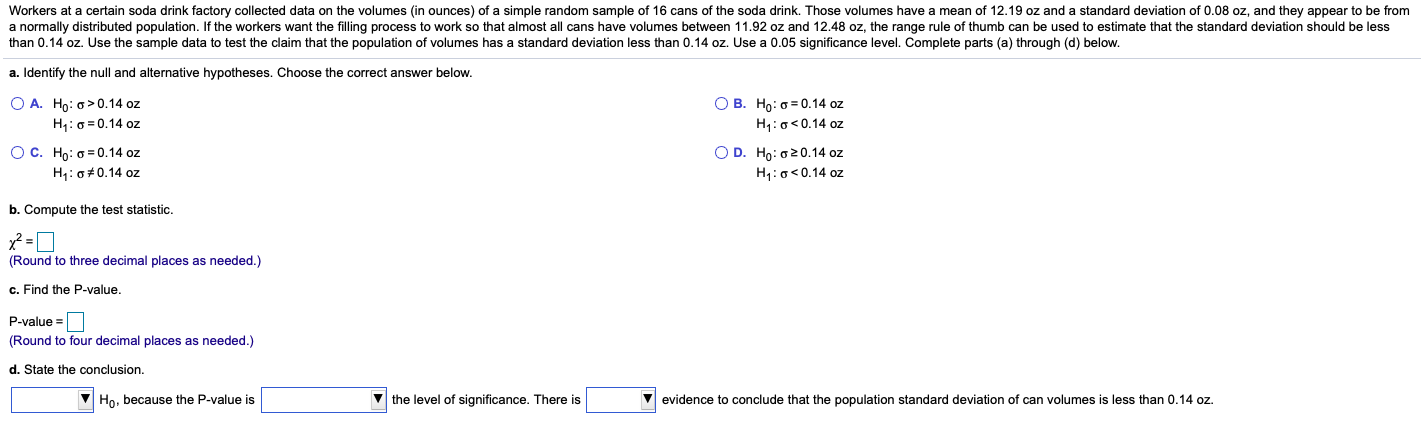Workers at a certain soda drink factory collected data on the volumes (in ounces) of a simple random sample of 16 cans of the soda drink. Those volumes have a mean of 12.19 oz and a standard deviation of 0.08 oz, and they appear to be from a normally distributed population. If the workers want the filling process to work so that almost all cans have volumes between 11.92 oz and 12.48 oz, the range rule of thumb can be...

• ### Workers at a certain soda drink factory collected data on the volumes (in ounces) of a...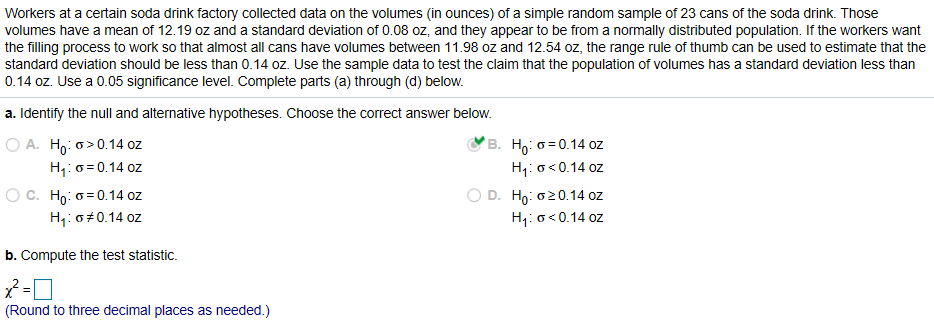Workers at a certain soda drink factory collected data on the volumes (in ounces) of a simple random sample of 23 cans of the soda drink. Those volumes have a mean of 12.19 oz and a standard deviation of 0.08 oz, and they appear to be from a normally distributed population. If the workers want the filling process to work so that almost all cans have volumes between 11.98 oz and 12.54 oz, the range rule of thumb can be...

• ### Workers at a certain soda drink factory collected data on the volumes (in ounces) of a...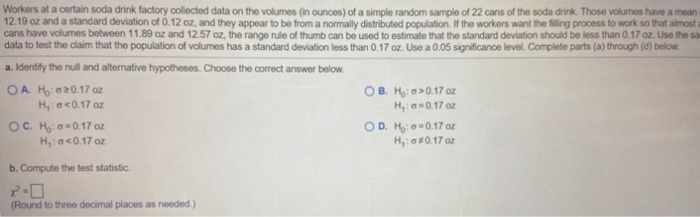Workers at a certain soda drink factory collected data on the volumes (in ounces) of a simple random sample of 22 cans of the soda drink. Those volumes have a mean 12.19 oz and a standard deviation of 0.12 oz, and they appear to be from a normally distributed population. If the workers want the filling process to work so that almost cans have volumes between 11.89 oz and 12.57 oz, the range rule of thumb can be used to...

• ### Workers at a certain soda drink factory collected data on the volumes (in ounces of a...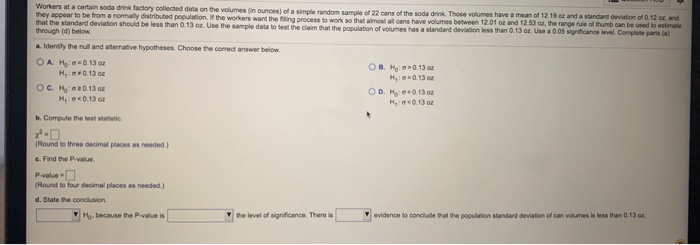Workers at a certain soda drink factory collected data on the volumes (in ounces of a simple random sample of 22 cans of the soda drink. Those volumes have a mean of 12.19 oz and a standard deviation of 0.12 r. and they appear to be from a normally distributed population. If the workers want the filing process to work so that almost alcans have volumes between 12.01 oz and 12 53 oz, the range rule of thumb can be...

• ### Soda six-packs Most soda cans list the volume of soda as 12 fluid ounces. As with...

Soda six-packs Most soda cans list the volume of soda as 12 fluid ounces. As with all process, some variation occurs when filling soda cans. Suppose that a company knows this and tries to over-fill cans a bit, so that the actual volume of soda in a can follows a normal distribution with mean 12.1 fluid ounces and standard deviation .15 fluid ounces. a) What proportion of soda cans filled by this process will contain less than 12 fluid ounces?...

• ### A study reports that teenagers drink an average of 22 ounces of soda per day with...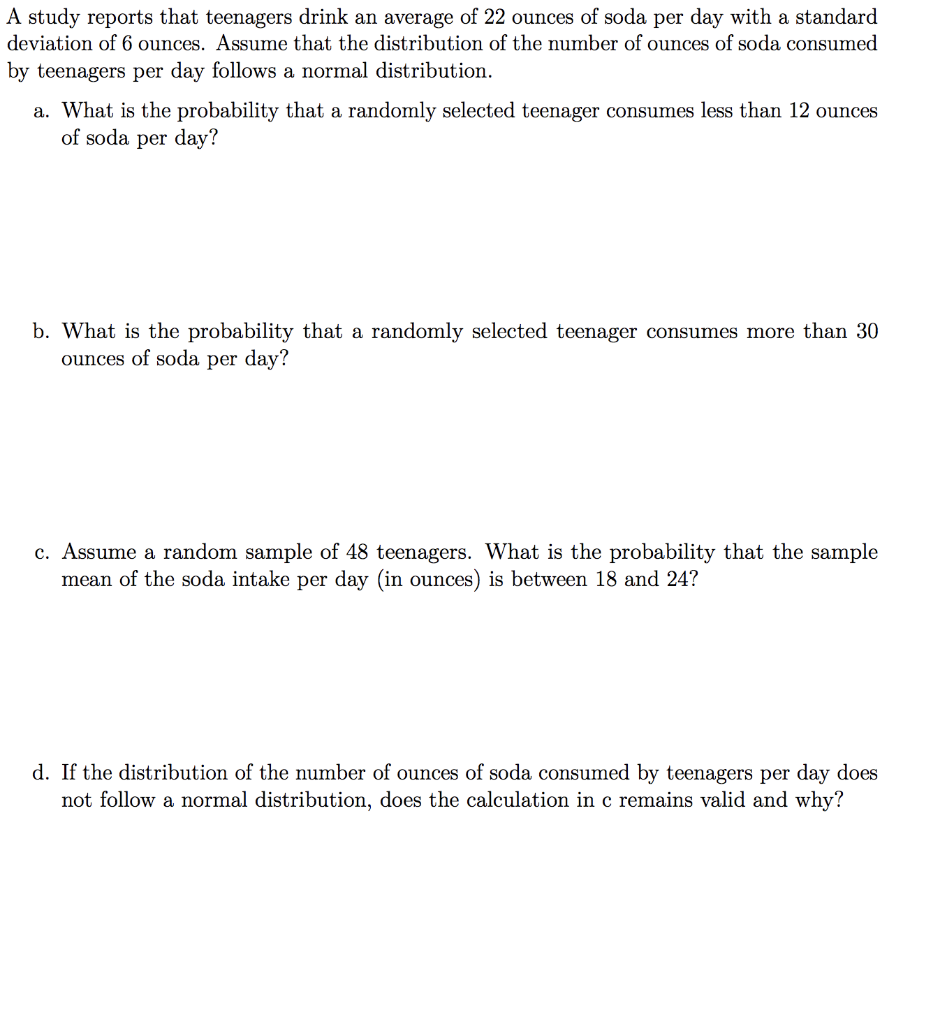A study reports that teenagers drink an average of 22 ounces of soda per day with a standard deviation of 6 ounces. Assume that the distribution of the number of ounces of soda consumed by teenagers per day follows a normal distribution. a. What is the probability that a randomly selected teenager consumes less than 12 ounces of soda per day? b. What is the probability that a randomly selected teenager consumes more than 30 ounces of soda per day?...

Free Homework App